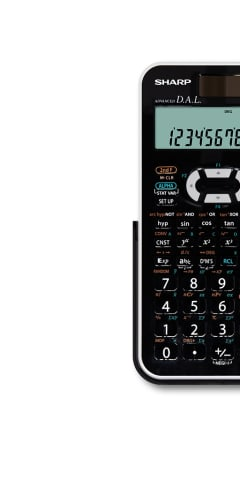EL506XBWH

# Scientific Calculator With 469 Functions

• 2 line LCD screen with upper 12 digit (5 x 7 dot matrix), lower 10 digit (mantissa) plus 2 digit (exponent).
• Up to 469 functions
• Twin power (solar cell and battery)
• Solver Function
• 9 Memories
• Fraction calculations
• Matrix Calculations
• Random number functions ( dice, coin, integer )
• Regression calculations ( 6 models )
POA## Scientific Calculator## LCD Display## Twin Powered## Protective Hard Cover• A scientific calculator designed for usage in science, engineering, and mathematics. Great for school/univeristy students or engineers
• Two-line display showing equation entered on top line and answer on the bottom line allowing users to check both the equation and answer at the same time. User can amend the equation if needed
• Twin powered solar cell and LR44 x 1 battery. Battery is secured with child-safe lock.
• Recall previous equations. 9 values can be stored in calculator, with 8 temporary memories and 1 independent memory.
• Store up to 469 scientific and statistical functions in calculator
• Hard, easy glide cover to protect calculator from scratches and for easy storage. Great for students to store their calculators in their school bags
• A portion of the equation is automatically memorised as a constant (Function Plus, Minus, Division, Multiplication), thus omitting redundant input. E.g. 2 x 5 = 10, pressing = will multiply by 5 again. This function is great for instances where you'll need to calculate the same equation with a different variable e.g. stocktake or estimating sales
• Ensures symbols and numbers of an equation to be entered as they are written. Instead of wasting energy on difficult calculator operations, users are free to concentrate on mathematical concepts. E.g. You want to input 10 + 2 x sin30= You push the keys in the same order as the actual equation.
• Allows you to enter numbers as fractions
• Allow equations to be entered without having to key the answer from previous equation repeatedly. This function enables calculation of a variable X in a given equation. Other variables (a, b, c, etc.) must already be defined in order to calculate X.
• Users are provided with transpose and cumulative matrix functions, among others. Up to four matrices can be saved in memory, each up to four rows by four columns in size.
• Performed in the statistics mode of the calculator. Six modes of regression calculations can be performed including Linear. Quadratic, Exponential, Logarithmic, Power and Inverse. Making this calculator great for high school student/university students and engineers.
• Simply enter numerical values following the interactive on-screen instructions, and you can find the answer easily of derivative and integral calculations. You don't have to re-enter the formula, and the calculation can be repeated as many times as needed.
• Conversions between numbers expressed in binary, octal, decimal and hexadecimal systems can be performed alongside the four basic arithmetic operations, calculations with parentheses and memory calculations using binary, octal, decimal, and hexadecimal numbers. In addition, the calculator can carry out the logical operations AND, OR, NOT, NEG, XOR and XNOR onbinary, octal and hexadecimal numbers
Calculator Types
Scientific Calculators
Display
2 Line LCD
Dimensions (WxHxD)
80 x 158 x 14 millimetre
Power Source
Solar cell & LR44 x 1 (battery)
Memory
9
Constant
Yes
Scientific & Stats function
469
Multi-line Playback
Yes
Chain Calculation
Yes
Fraction Key
Yes
Hard Cover
Yes, slide-on